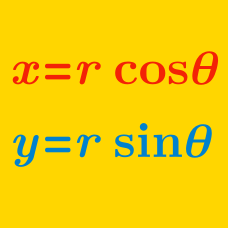Algebra

# Polar Coordinates - Multiplication

Let $z = 3(\cos(15 ^\circ) + i\sin(15 ^\circ)),$ $w = 5(\cos(54 ^\circ)+i\sin(54 ^\circ)).$ Then $zw$ can be expressed as $r(\cos \alpha ^ \circ + i\sin\beta ^ \circ )$, where $r$ is a real number, $0 \leq \alpha \leq 90$ and $0 \leq \beta \leq 90$. What is $r+\alpha+\beta$?

Let $z_1$ and $z_2$ be complex numbers such that $z_1 = 15 \left( \cos \frac{5}{12}\pi + i \sin \frac{5}{12}\pi \right),$ $z_2 = 2 \left( \cos \frac{1}{12}\pi + i \sin \frac{1}{12}\pi \right).$ The product $z_1 z_2$ can be expressed as $a + bi,$ where $a$ and $b$ are real numbers. What is the value of $a+b?$

Details and assumptions

$i$ is the imaginary number satisfying $i^2 = -1$.

Consider the complex numbers \begin{aligned} z_1 &= 5+5\sqrt{3}i, \\ z_2 &= 3\left(\cos \frac{\pi}{6}+i\sin \frac{\pi}{6}\right). \end{aligned} If the product $z_1z_2$ can be expressed as $a+bi$, where $a$ and $b$ are real numbers, what is $a+b$?

Let $z_1, z_2$ and $z_3$ be complex numbers such that \begin{aligned} z_1 &= 2 \left( \cos \frac{1}{12}\pi + i \sin \frac{1}{12}\pi \right), \\ z_2 &= 7 \sqrt{3} \left( \cos \frac{1}{12}\pi + i \sin \frac{1}{12}\pi \right), \\ z_3 &= 9 \left( \cos \frac{1}{6}\pi + i \sin \frac{1}{6}\pi \right). \end{aligned} If the product $z_1 z_2 z_3$ can be expressed as $a + bi,$ where $a$ and $b$ are real numbers, what is $\frac{b^2}{a^2}$?

Let $z_1$ and $z_2$ be complex numbers such that $z_1 = 10 \left( \cos \frac{1}{24}\pi + i \sin \frac{1}{24}\pi \right),$ $z_2 = 5 \sqrt{2} \left( \cos \frac{5}{24}\pi + i \sin \frac{5}{24}\pi \right).$ The product $z_1 z_2$ can be expressed as $a + bi,$ where $a$ and $b$ are real numbers. What is the value of $a+b$?

Details and assumptions

$i$ is the imaginary number that satisfies $i^2 = -1$.

×